GFG App
Open AppBrowser
Continue

# MongoDB – Equality Operator \$eq

MongoDB provides different types of comparison operators and an equality operator(\$eq) is one of them. The equality operator( \$eq ) is used to match the documents where the value of the field is equal to the specified value. In other words, the `\$eq` operator is used to specify the equality condition.

Important Points:

• If the given value is a document, then the order of the fields in the document is important.
• If the given value is an array, then MongoDB matches the documents where the field contains an element that exactly matches the specified array.

Syntax:

```{field: {\$eq: value}}
or
{field: value}

```

In the following examples, we are working with:

Database: GeeksforGeeks
Collection: employee
Document: five documents that contain the details of the employees in the form of field-value pairs.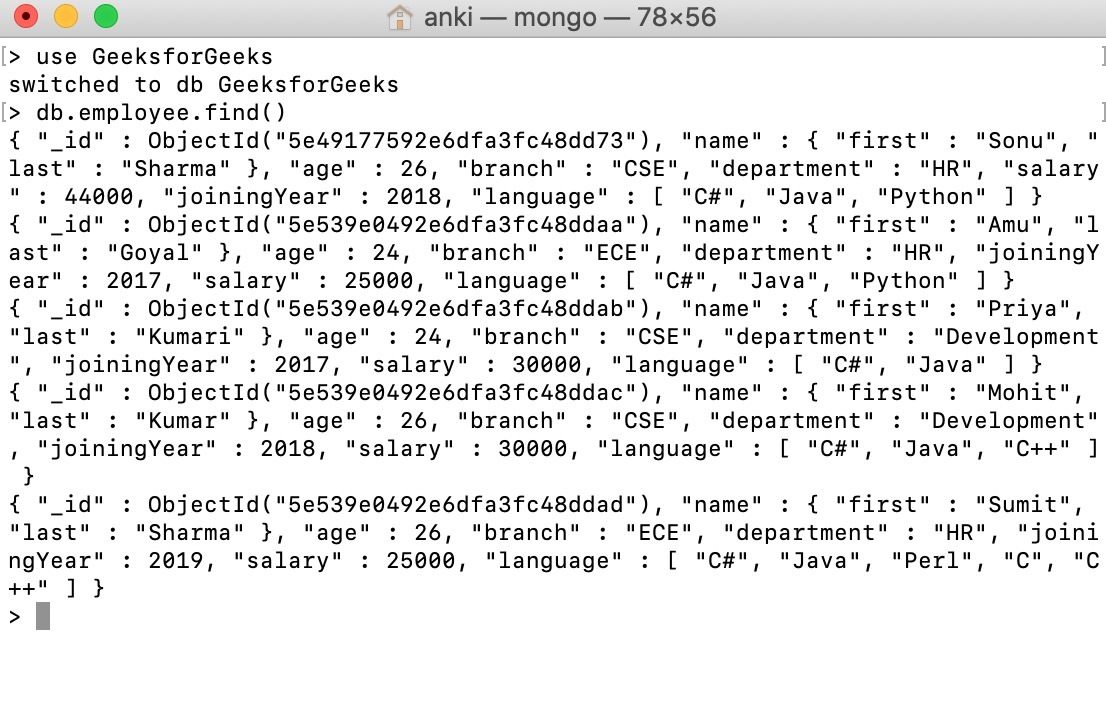Example #1:
In this example, we are selecting those documents where the value of the salary field is equal to 30000.

 `db.employee.find({salary: {\$eq: ``30000``}}).pretty() `

It is equivalent to –

 `db.employee.find({salary: ``30000``}).pretty() `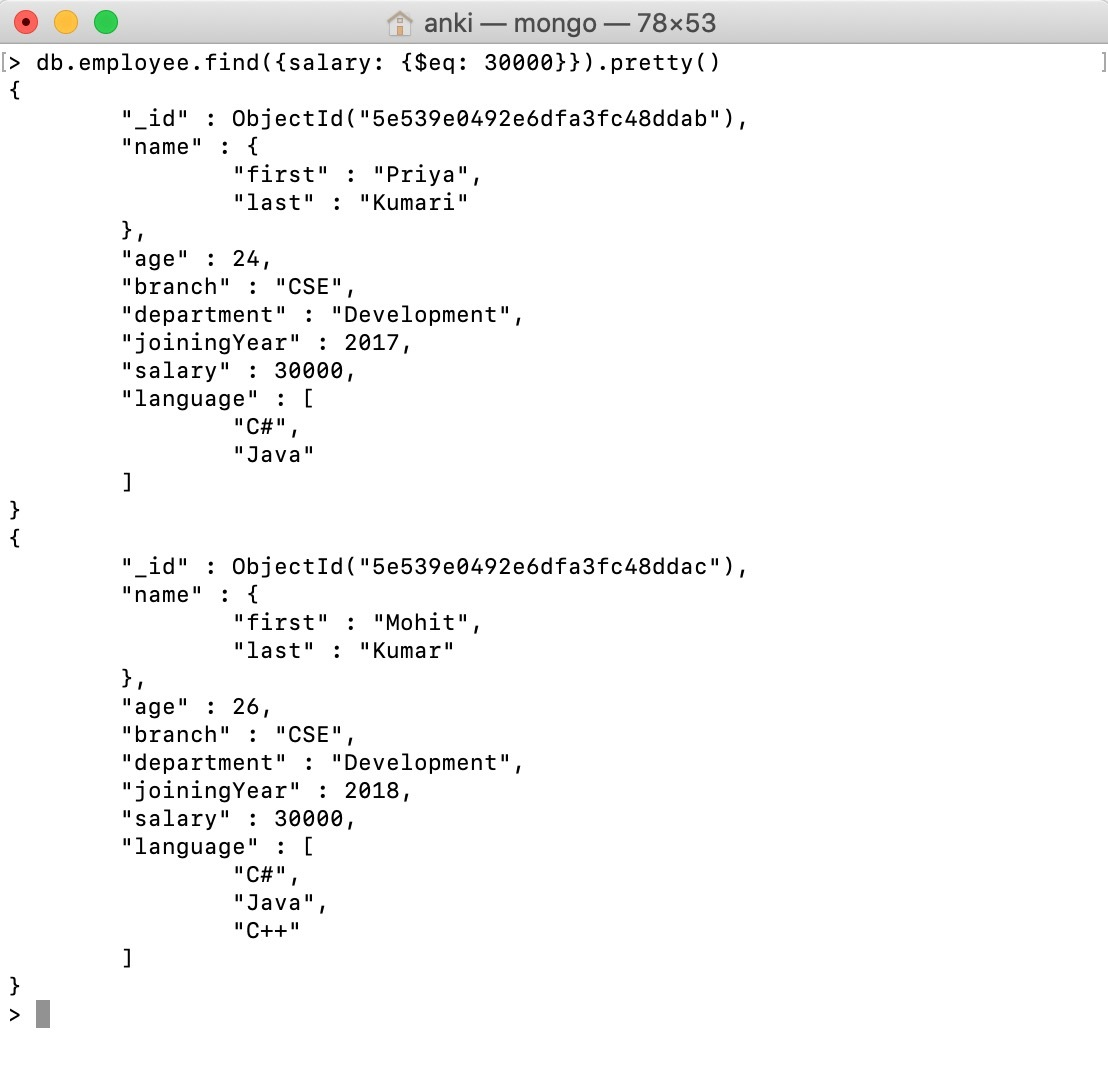Example #2:
In this example, we are selecting those documents where the first name of the employee is equal to Amu. or in other words, in this example, we are specifying conditions on the field in the embedded document using dot notation.

 `db.employee.find({``"name.first"``: {\$eq: ``"Amu"``}}).pretty() `

It is equivalent to:

 `db.employee.find({``"name.first"``: ``"Amu"``}).pretty() `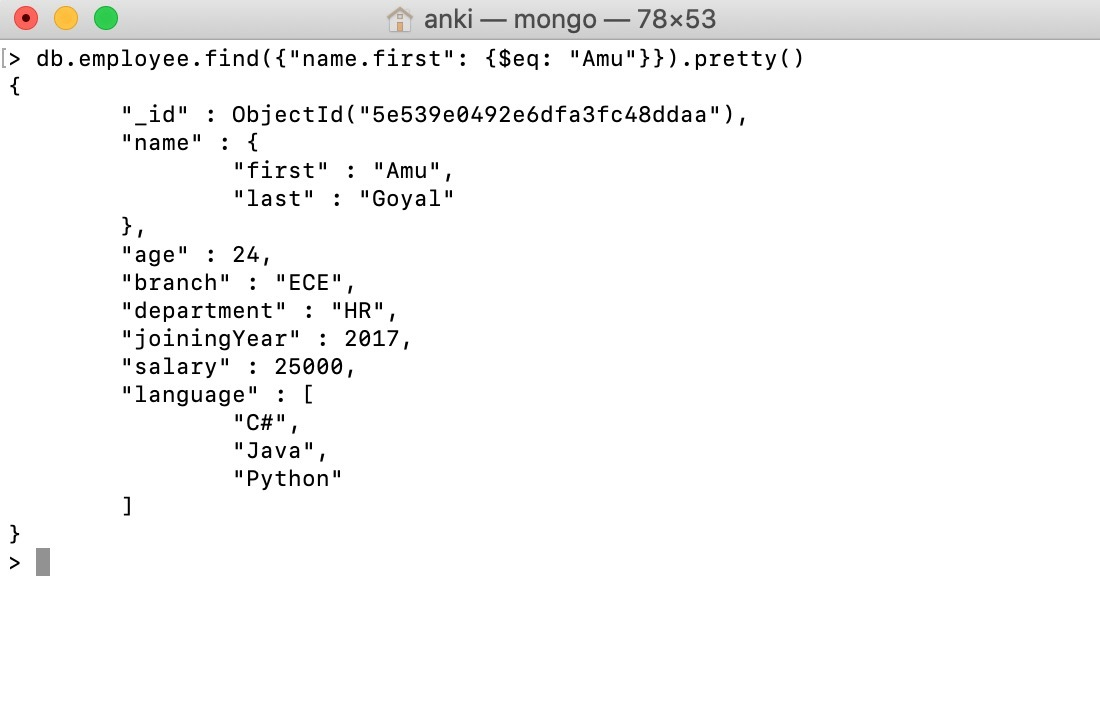Example #3:
In this example, we are selecting those documents where the language array contains an element with value “C++”.

 `db.employee.find({language: {\$eq: ``"C++"``}}).pretty() `

It is equivalent to:

 `db.employee.find({language: ``"C++"``}).pretty() `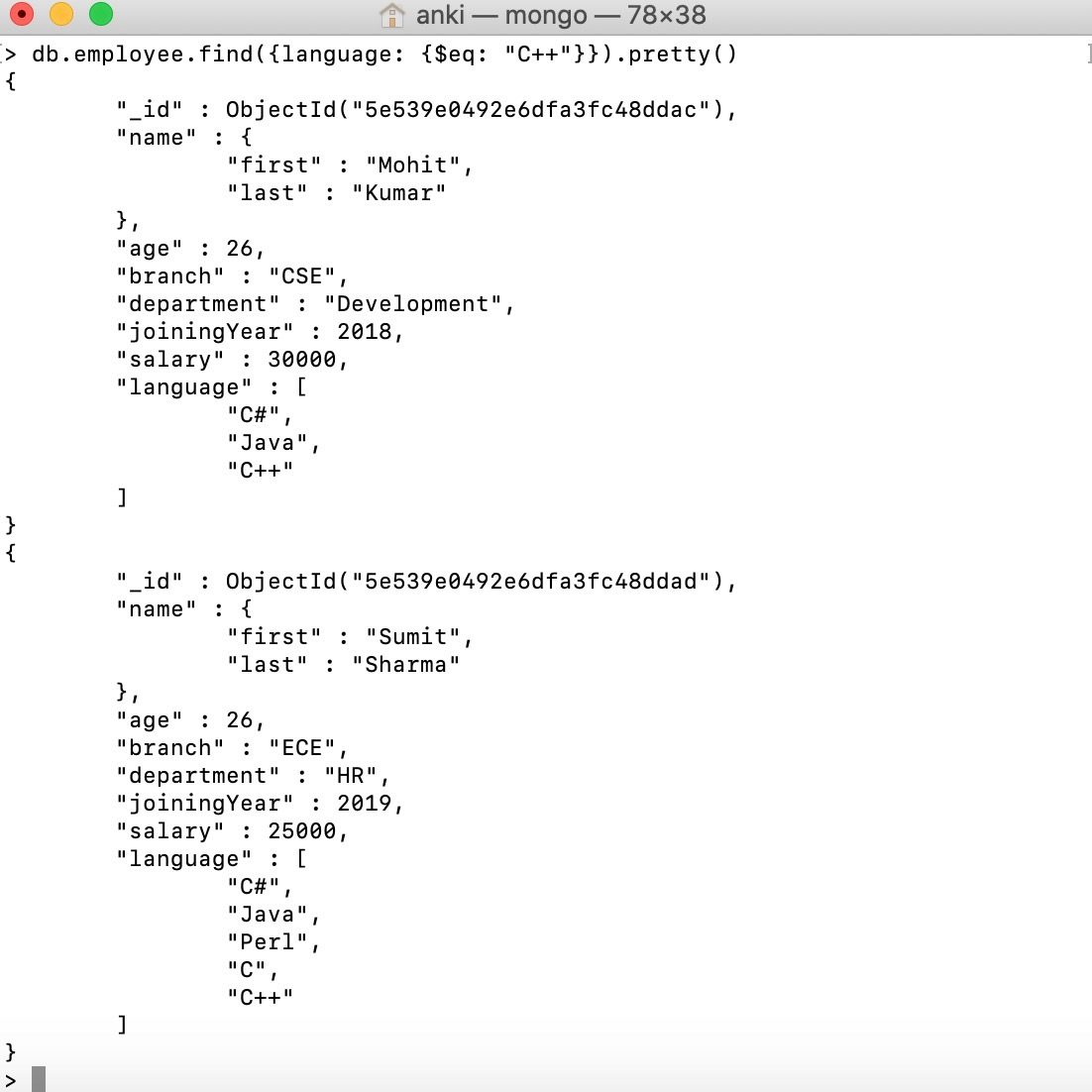Example #4:
In this example, we are selecting those documents where the language array is equal to the specified array.

 `db.employee.find({language: {\$eq: [``"C#"``, ``"Java"``]}}).pretty() `

It is equivalent to:

 `db.employee.find({language:[``"C#"``, ``"Java"``]}).pretty() `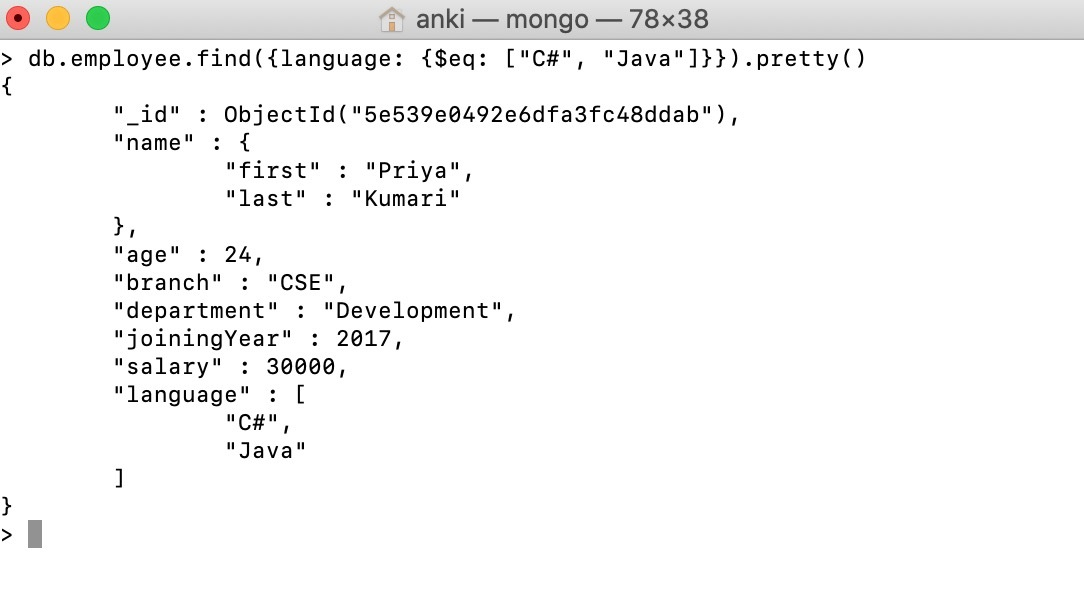My Personal Notes arrow_drop_up